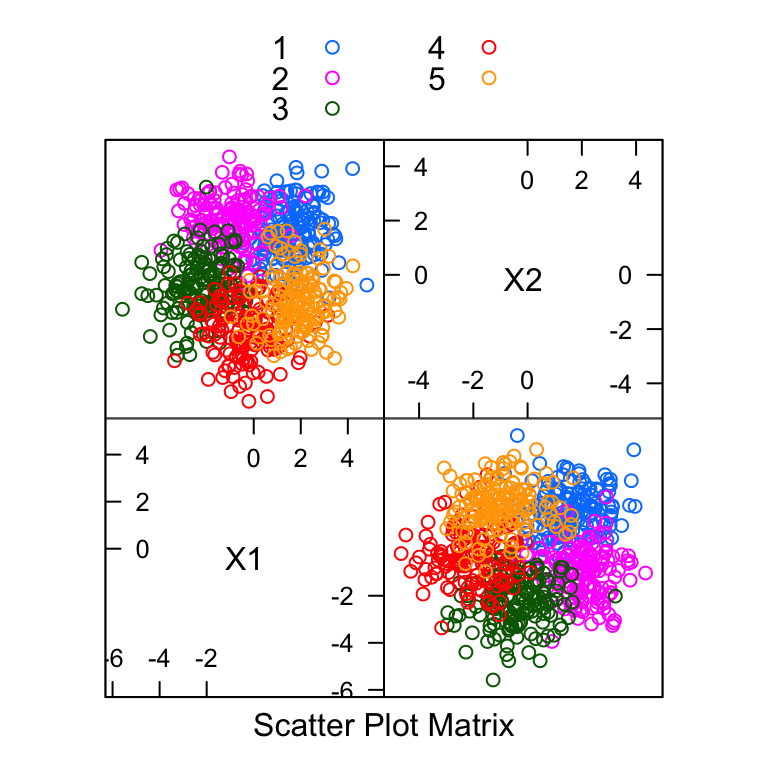Please see the homework instructions document for detailed instructions and some grading notes. Failure to follow instructions will result in point reductions.

You should use the caret package and training pipeline to complete this homework. Any time you use the train() function, first run set.seed(1337).

library(caret)
library(mlbench)

# Exercise 1 (Regression with caret)

[10 points] For this exercise we will train a number of regression models for the Boston data from the MASS package. Use medv as the response and all other variables as predictors. Use the test-train split given below. When tuning models and reporting cross-validated error, use 5-fold cross-validation.

data(Boston, package = "MASS")
set.seed(42)
bstn_idx = createDataPartition(Boston$medv, p = 0.80, list = FALSE) bstn_trn = Boston[bstn_idx, ] bstn_tst = Boston[-bstn_idx, ] Fit a total of five models: • An additive linear regression • A well tuned $$k$$-nearest neighbors model. • Do not scale the predictors. • Consider $$k \in \{1, 5, 10, 15, 20, 25\}$$ • Another well tuned $$k$$-nearest neighbors model. • Do scale the predictors. • Consider $$k \in \{1, 5, 10, 15, 20, 25\}$$ • A random forest • Use the default tuning parameters chosen by caret • A boosted tree model • Use the provided tuning grid below gbm_grid = expand.grid(interaction.depth = c(1, 2, 3), n.trees = (1:20) * 100, shrinkage = c(0.1, 0.3), n.minobsinnode = 20) Provide plots of error versus tuning parameters for the two $$k$$-nearest neighbors models and the boosted tree model. Also provide a table that summarizes the cross-validated and test RMSE for each of the five (tuned) models. # Exercise 2 (Clasification with caret) [10 points] For this exercise we will train a number of classifiers using the training data generated below. The categorical response variable is classes and the remaining variables should be used as predictors. When tuning models and reporting cross-validated error, use 10-fold cross-validation. set.seed(42) sim_trn = mlbench::mlbench.2dnormals(n = 750, cl = 5) sim_trn = data.frame( classes = sim_trn$classes,
sim_trn$x ) caret::featurePlot(x = sim_trn[, -1], y = sim_trn$classes,
plot = "pairs",
auto.key = list(columns = 2))Fit a total of four models:

• LDA
• QDA
• Naive Bayes
• Regularized Discriminant Analysis (RDA)
• Use method rda with caret which requires the klaR package
• Use the default tuning grid

Provide a plot of acuracy versus tuning parameters for the RDA model. Also provide a table that summarizes the cross-validated accuracy and their standard deviations for each of the four (tuned) models.

# Exercise 3 (Concept Checks)

[1 point each] Answer the following questions based on your results from the three exercises.

## Regression

(a) What value of $$k$$ is chosen for KNN without predictor scaling?

(b) What value of $$k$$ is chosen for KNN with predictor scaling?

(c) What are the values of the tuning parameters chosen for the boosted tree model?

(d) Which method achieves the lowest cross-validated error?

(e) Which method achieves the lowest test error?

## Classification

(f) What are the values of the tuning parameters chosen for the RDA model?

(g) Based on the scatterplot, which method, LDA or QDA, do you think is more appropriate? Explain.

(h) Based on the scatterplot, which method, QDA or Naive Bayes, do you think is more appropriate? Explain.

(i) Which model achieves the best cross-validated accuracy?

(j) Do you believe the model in (i) is the model that should be chosen? Explain.